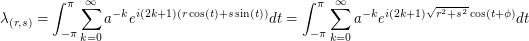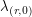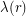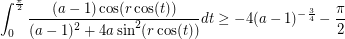### Solution (continued)

We see that the eigenvalue of the eigenfunctionis given byfor an appropriately chosen. Thus we need only actually consider, which we from now on denote. Then some calculation gives us that the integral isWe can show that (and this will suffice) that whenis near,